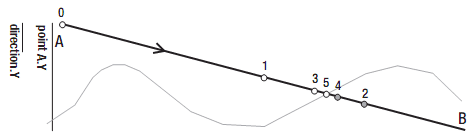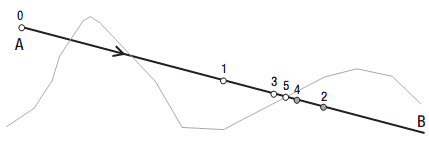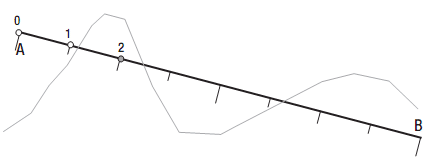# 5.10 计算光标与地形的碰撞点：表面拾取

## 工作原理

private Ray GetPointerRay(Vector2 pointerPosition)
{
Vector3 nearScreenPoint = new Vector3(pointerPosition.X, pointerPosition.Y, 0);
Vector3 farScreenPoint = new Vector3(pointerPosition.X, pointerPosition.Y, 1);

Vector3 near3DWorldPoint = device.Viewport.Unproject(nearScreenPoint, moveCam.ProjectionMatrix, moveCam.ViewMatrix, Matrix.Identity);
Vector3 far3DWorldPoint = device.Viewport.Unproject(farScreenPoint, moveCam.ProjectionMatrix, moveCam.ViewMatrix, Matrix.Identity);

Vector3 pointerRayDirection = far3DWorldPoint - near3DWorldPoint;
Ray pointerRay = new Ray(near3DWorldPoint, pointerRayDirection);

return pointerRay;
} 

### 二分法搜索### 二分法搜索方法

1. 将射线方向一分为二。
2. 将结果方向添加到当前点获取下一个点。
3. 如果下一个点仍在地形上方，将这个点作为当前点。

private Vector3 BinarySearch(Ray ray)
{
float accuracy = 0.01f;

float heightAtStartingPoint = terrain.GetExactHeightAt(ray.Position.X, -ray.Position.Z);
float currentError = ray.Position.Y - heightAtStartingPoint;

while (currentError > accuracy)
{
ray.Direction /= 2.0f;
Vector3 nextPoint = ray.Position + ray.Direction;
float heightAtNextPoint = terrain.GetExactHeightAt(nextPoint.X, -nextPoint.Z);

if (nextPoint.Y > heightAtNextPoint)
{
ray.Position = nextPoint;
currentError = ray.Position.Y - heightAtNextPoint;
}
}
return ray.Position;
} 

pointerPos = BinarySearch(pointerRay);

### 二分法搜索的问题### 线性搜索LinearSearch方法以AB间的射线为参数，将这根射线分成相同的线段，返回对应哪段发生碰撞的一部分射线：

private Ray LinearSearch(Ray ray)
{
ray.Direction /= 300.0f;

Vector3 nextPoint = ray.Position + ray.Direction;
float heightAtNextPoint = terrain.GetExactHeightAt(nextPoint.X, -nextPoint.Z);

while (heightAtNextPoint < nextPoint.Y)
{
ray.Position = nextPoint;
nextPoint = ray.Position + ray.Direction;
heightAtNextPoint = terrain.GetExactHeightAt(nextPoint.X, -nextPoint.Z);
}
return ray;
} 

Ray shorterRay = LinearSearch(pointerRay);
pointerPos = BinarySearch(shorterRay); 

### 指定程序的优化

private Ray ClipRay(Ray ray, float highest, float lowest)
{
Vector3 oldStartPoint = ray.Position;
float factorH = -(oldStartPoint.Y-highest) / ray.Direction.Y;
Vector3 pointA = oldStartPoint + factorH * ray.Direction;
float factorL = -(oldStartPoint.Y-lowest) / ray.Direction.Y;
Vector3 pointB = oldStartPoint + factorL * ray.Direction;
Vector3 newDirection = pointB - pointA;

return new Ray(pointA, newDirection);
} 

## 代码

protected override void Update(GameTime gameTime)
{

this.Exit();

MouseState mouseState = Mouse.GetState();
KeyboardState keyState = Keyboard.GetState();

Vector2 pointerScreenPos = new Vector2(mouseState.X, mouseState.Y);
Ray pointerRay = GetPointerRay(pointerScreenPos);
Ray clippedRay = ClipRay(pointerRay, 30, 0);
Ray shorterRay = LinearSearch(clippedRay);
pointerPos = BinarySearch(shorterRay);

base.Update(gameTime);
} 

private Ray ClipRay(Ray ray, float highest, float lowest)
{
Vector3 oldStartPoint = ray.Position;
float factorH = -(oldStartPoint.Y-highest) / ray.Direction.Y;
Vector3 pointA = oldStartPoint + factorH * ray.Direction;
float factorL = -(oldStartPoint.Y-lowest) / ray.Direction.Y;
Vector3 pointB = oldStartPoint + factorL * ray.Direction;
Vector3 newDirection = pointB - pointA;

return new Ray(pointA, newDirection);
} 

private Ray LinearSearch(Ray ray)
{
ray.Direction /= 50.0f;
Vector3 nextPoint = ray.Position + ray.Direction;
float heightAtNextPoint = terrain.GetExactHeightAt(nextPoint.X, -nextPoint.Z);

while (heightAtNextPoint < nextPoint.Y)
{
ray.Position = nextPoint;
nextPoint = ray.Position + ray.Direction;
heightAtNextPoint = terrain.GetExactHeightAt(nextPoint.X, -nextPoint.Z);
}

return ray;
} 

private Vector3 BinarySearch(Ray ray)
{
float accuracy = 0.01f;

float heightAtStartingPoint = terrain.GetExactHeightAt(ray.Position.X,-ray.Position.Z);
float currentError = ray.Position.Y - heightAtStartingPoint;

int counter = 0;
while (currentError > accuracy)
{
ray.Direction /= 2.0f;
Vector3 nextPoint = ray.Position + ray.Direction;
float heightAtNextPoint = terrain.GetExactHeightAt(nextPoint.X, -nextPoint.Z);

if (nextPoint.Y > heightAtNextPoint)
{
ray.Position = nextPoint;
currentError = ray.Position.Y - heightAtNextPoint;
}

if (counter++ == 1000)
break;
}
return ray.Position;
}

2006 - 2023，推荐分辨率1024*768以上，推荐浏览器Chrome、Edge等现代浏览器，截止2021年12月5日的访问次数：1872万9823 站长邮箱